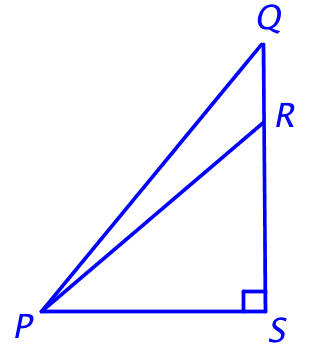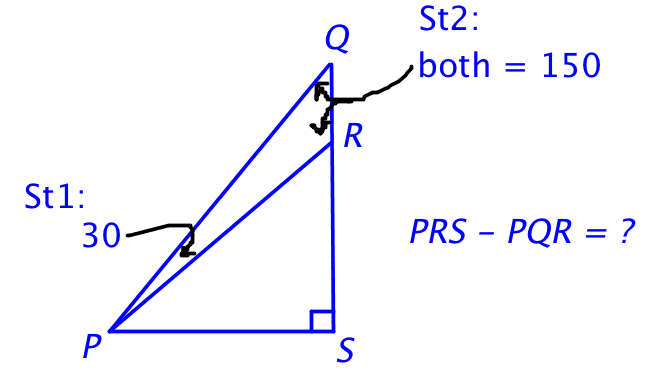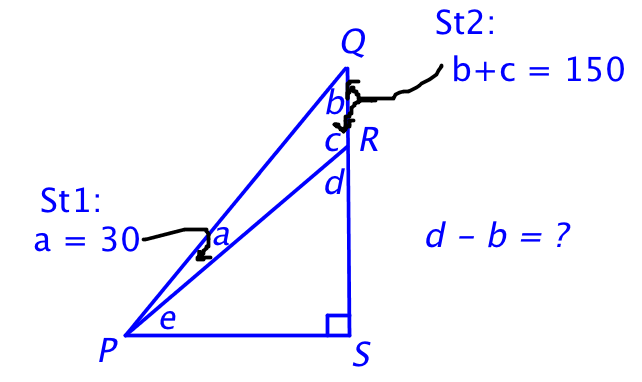# How to Become a Great GMAT Guesser - Part 2

by , Apr 20, 2016Welcome to part 2 in our guessing series. You know youre going to have to guess on the GMAT. The only real questions are when and how?

Last time, we talked about how to guess on Average Speed questions. This time, Ive got a Data Sufficiency problem for you from the free GMATPrep tests. The first time you try it, pretend you have just 60 seconds until the timer is going to run out on your test. What are you going to guess? (You can try it for real after that. :) )*In the figure shown, the measure of angle PRS is how many degrees greater than the measure of angle PQR?

(1) The measure of angle QPR is 30.

(2) The sum of the measures of angles PQR and PRQ is 150.

On your first pass, what did you guess? And why did you guess that?

If your answer is, I dont know. I didnt figure anything out, so I just guessed my favorite letter, then my response is, Great job! Seriously. You did make a guess. Thats the most important partit can be hard to force yourself to let go. Ill only be disappointed if you didnt guess at all, since your first assignment was to make some kind of guess. (Did you not guess? Guess right now. Even if its your favorite letter. Say it out loud. Do it!)

Okay. Lets do this thing. Normally, of course, youd draw the shape on your scrap paper. But you might skip this step if you realize that youre running out of time and are going to have to guess. Instead, just use what you see on screen. (Also: remember that DS diagrams are not necessarily drawn to scale.)

Now, what are they asking? The difference between those two angles ... but, wow, they only told me that one angle is a right angle. Nothing else.

Okay, Ill just look at the statements to see whether anything jumps out at me. Otherwise, Im picking my favorite letter.

(1) The measure of angle QPR is 30.

Ive got nothing. If I had to guess right now, Id say nope. Only two angles, this one and the right angle? Not enough.

(2) The sum of the measures of angles PQR and PRQ is 150.

And still nothing. Its possibly a little better than statement (1), I guess, but it still doesnt seem like enough, right?Hmm. Something did catch my eye, though. 30 and 150 add up to 180. That makes sense of courseits a triangle, so it has to add up to 180.

But wait! Dont dismiss that thought so fast. Something important just happened.

If angle QPR is 30 degrees, then you actually already know that the sum of the other two is 150. Alternatively, if you first know that the sum of the other two is 150, then you know that the third angle, QPR, must be 30.

In other words, each separate statement is telling you exactly the same information: angle QPR is 30 and the sum of the other two is 150. Thats a crucial detail! If each statement says exactly the same thing, then either each one works by itself (in which case the answer is D) or neither one works, in which case they also cant work when you combine them (since you dont get any new information by combining them). In that second case, the answer is E.

In other words:

If the two DS statements tell you exactly the same information,

then the answer must be either (D) or (E). Always.

Boom. Guess one of those two and move on.

And one last bit of advice. When you know that you really dont have any idea whats going on in the problem, guess the answer that seems less likely. So, even though my initial thoughts were that theres no way the statements could work alone, Id still guess (D), for this simple reason: other people are going to guess, too. And those people are going to guess (E) because, wow, does this not look like enough info. It seems really obvious!

But the GMAT cant afford for the correct answer to be the obvious answer. Too many people would guess correctly and that would mess up the test.

So I'd guess (D) and, yes, that's the correct answer on this one. How in the world is that possible? Take a look.

First, they asked for the difference between two angles, not the actual degree measures of the individual angles. Plus, they seem obviously not to give you enough info. Its true that theres not enough info to find the individual degree measuresbut they didnt ask you to find those. So thats a good clue that you probably really can solve for the difference.

First, write out what you want to find:

PRS PQR = ?

Hang on a second. Its pretty annoying to use the three letters to label each angle. And I think that might lead to a careless mistake. Lets re-label.Much better. Okay, how to find d b?

As discussed earlier, if QPR (now a) is 30, then the sum of PQR and PRQ (now b and c) must be 150:

a = 30

b + c = 150

That takes care of one of the three triangles in this diagram. What about the other two?

In the other small triangle, the three angles also add up to 180. One angle is a right angle, so the other two angles have to add up to 90:

d + e = 90

You can do something similar for the big triangle:

(a + e) + b = 90

(30 + e) + b = 90

Now, how can you rearrange all of that to get only d and b? Lets start with:

d + e = 90

The d is already there. Now, how to get rid of the e and get b instead? Take the equation with e and b in itand rearrange to isolate e:

(30 + e) + b = 90

e = 60 b

Finally, substitute:

d + (60 b) = 90

We can find d b. (Dont, of course! Its Data Sufficiency: stop when you know you can do it.)

Statement (1) works by itself. And since statement (2) provides the same info, it must work by itself, too.